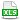HomeTroubleshooting@RISK for Excel: SimulationSum of Percentiles Differs from Percentile of the Sum

# 4.22. Sum of Percentiles Differs from Percentile of the Sum

Applies to: @RISK, all releases

In my model, I have a set of inputs. I strike a total, and that is my output. But I also compute the 95th percentiles of the inputs and the 95th percentile of the output using RiskPercentile( ) or RiskPtoX( ). Why does a percentile of the output not equal the sum of that percentile of the inputs?

This is normal behavior. Unless your inputs are correlated with a coefficient of 1, they won't all be at a given percentile in the same iteration. In most iterations, some inputs will be above any particular percentile and some will be below. When you add them all up, the highs and lows tend to balance out, so the 95th percentile of the sum will be closer to the mean than the sum of the 95th percentiles of the inputs.

It's not just percentiles — any statistic other than the mean will have a similar issue. This is a statistical feature of combining probability distributions, and not any kind of problem in @RISK or in your model.

For a related issue, see Static Value of Output Differs from Simulated Mean.

Okay then, which one is right?

They're both right, but they mean different things. Please open the example that's attached to this article.

It's almost always more useful to take the percentile of the sum, the green figure in the example. For example, that tells you how much to set aside in reserves if you want 95% confidence that you've set aside enough. There's a 95% chance that the total of all eight risks will turn out to be less than that figure. Sure, some risks may be above their individual 95th percentiles, but most will be below their 95th percentiles. The green figure is the realistic measure of your total risk.

Suppose you want a sort of worst-case scenario? What if every risk happens to come in at its 95th percentile? (Of course that is very unlikely, only about one chance in 25,600,000,000.) The total of the eight individual 95th percentiles is the orange figure in the P95 column. If you set aside that larger figure as a reserve, you're making a reserve for a situation with only 0.004% of a millionth of a chance of occurring.

Additional keywords: Maximum of sum doesn't equal sum of maximums; Minimum of sum doesn't equal sum of minimums

Last edited: 2018-04-19

•KB1296_Percentiles.xlsx